> > To measure an angle

To measure an angle
1. Click Analysis and then, in the Measure group, click the arrow next to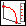Angle.
2. ClickAngle,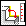Angle between Edges,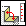Angle between Faces,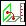Angle between Workplanes, or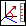Angle between Directions to select a measuring angle option. The Measure Output and Angle dialog boxes open.
3. Select a measuring option:
Click Angle between Edges. Select the edge whose angle you want to measure.
Click Angle between Faces. Click the face whose angle you want to measure.
Click Angle between Workplanes. Select the workplane whose angle you want to measure.
Click Angle between Directions. Select the direction whose angle you want to measure (using the options in the Direction 3D CoPilot tool).
4. Select the reference object, using one of the following ways:
To calculate the angle relative to another edge, click the edge.
To calculate the angle relative to a face's normal direction, click Face and click the face.
To calculate the angle relative to a workplane's normal direction, click Workplane and specify the workplane.
To calculate the angle relative to a direction, click Direction and specify the direction (using the options in the Direction 3D CoPilot tool).
5. Creo Elements/Direct Modeling displays graphical feedback representing the angle and displays the angle value in the Angle Output field.
6. You can manipulate the angle in the following ways:
To reverse the first object's direction, click Rev 1st Dir.
To reverse the second object's direction, click Rev 2nd Dir.
To reverse the first object's direction with the second, and the second object's direction with the first, click Switch Dir.
To measure the complement angle, click Complement.
7. Creo Elements/Direct Modeling updates the graphical feedback representing the angle and displays the new angle value in the Angle Output field.
8. Click the green arrow button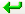to send the measurement to a command.
9. Click the green arrow and calculator button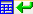to send the measurement to the calculator.